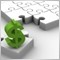EA - iFractal (different period than the current one)24

Greetings,

I need some help from you guys.

Is it possible to use iFractals with different period than the current one?

I use this to get latest low / high line , but it only worlk is the period used on chart is the same as the parameter.

Can I get the latest fractals from other timelines with iFractal?

Thanks.

int getFractal(int dir)

{
for(int i=0;i<2000;i++)
{
double val = iFractals(Symbol(),PERIOD_M15,dir,i);
if(val != 0)
{
if(dir == 1) f_h = val;
else if(dir == 2) f_l = val;
return;
}
}
}8783

puiucristian:

int getFractal(int dir)

{
for(int i=0;i<2000;i++)
{
double val = iFractals(Symbol(),PERIOD_M15,dir,i);
if(val != 0)
{
if(dir == 1) f_h = val;
else if(dir == 2) f_l = val;
return;
}
}
}
Change the highlighted part of your code to whatever timeframe you want